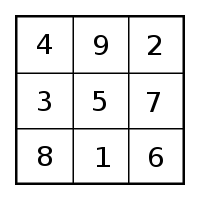## Four Hundred Fortieth Post Defines Genesis 1:1

Four Hundred Fortieth Post Defines Genesis 1:1. The explanation involves  a few steps. We begin with the 27 x 27 number square.  Notice the straight track. The track is the line of the diagonal from numbers 352 to 378. Also notice, it includes numbers that define the 365 days of the solar year, We also have the 354 days of the lunar year. Please note: 365 is the exact  central number of the number square.  Earth days sit directly at the center. Then take a compass to this square. Draw a circle from center point 365. Make the circumference pass through square 354.  You have a model for the Moon rotating around the Sun. The number of days it takes for each are specified.  My source for the featured picture is Magic Squares and Cubes by W.S. Andrews. Antiquity and knowledge of number squares went hand in hand.

lunar calendar is a calendar based upon the monthly cycles of the Moon‘s phases (synodic months), in contrast to solar calendars, whose annual cycles are based only directly upon the solar year. Each lunation is approximately ​29 12 days. it Is common for the months of a lunar calendar to alternate between 29 and 30 days. A lunar year, is 354.367056 days. I have two internal links. If you have the time, you can read them for background.

## A Code for the Cosmos, Sciences and Arts

##### Creation: Phi & Exponential factor are in Genesis 1:1 – DSO Works   -Below is a short quote from the Creation blog. It points to the basic use of number 27. In the past, numbers squares crossed borders into geometry and vice versa. Buckminster Fuller would call this “synergy.” Today we do not think of numbers in this manner. Our way of thinking believes that numbers are pure and abstract in themselves. Not so in the past!
• The 1st sentence of Genesis equals in Hebrew is 2701. One (#1) was often added by tradition of Colel. “Colel” represented the singular Deity,  Here is the source of the hidden 27 of Genesis . The simplest number square is the  “grain of mustard seed. Ancients named it the square of Saturn. Today we call it the 3 x 3 number square

### Why is this called the four hundred fortieth post?3 x 3 number square that was once associated with Saturn.
• Add the numbers around the central 5. The eight numbers total 40. Then add them two at the time overlapping the numbers: 49 + 92 + 27 + 76 + 61 + 18 + 83 + 34 = 440. This number is my key blog phrase – four hundred fortieth post. .
• Now take the numbers three at the time. Overlap the four sets of triple numbers around the perimeter as: 492 + 276 + 618 +  834 = 2,220. They come to the same totals when added in reverse. Let (P) stand for perimeter.
• Then (p-1)   is 40 + (P-2)  is 440 + ( P-3) is 2,220  = 2700. Add one for the Deity and you have 2701. They knew the 27 x 27 number square held our planetary timing numbers. Zero was not recognized as an independent number in antiquity. It was considered synthetic.

A lost civilization synergetically set out 2700 total of these 3 perimeters. They knew the 27 x 27 number square held our planetary timing numbers.They ascribed it to the 27 x 27  number square’s diagonal of the featured picture.